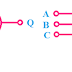## AND Gate

The AND Gate is a logic gate which creates an LOW(0) output signal when any one input signal is LOW(0) or in other words, if the all input signal is HIGH(1) then the AND Gate creates HIGH(1) output signal. We are Going to know AND Gate Truth Table, Internal Circuit Design, Symbol.

The AND gate perform logical AND Operation in digital electronics. Basically, the AND has three terminal two input terminals and one output terminal. The AND gates are comes in form of ICs.

### The Concept and operation of AND Gate:

In digital electronics, there are only two types of operation is done ON or OFF. The AND Gates also does the same. You can say it act as a switch which will ON or OFF according to some conditions. If you see the truth table of the AND gate then you will understand the operation of the AND Gate.

As the AND gate has two input terminal so we can apply two digital signals together. The operation of the AND Gate according to the truth table is given below,

1.      When input A and input B both are Low(0) then the AND Gate create Low(0) output signal.
2.      When input A is Low(0) and the input B is High(1) then the AND Gate create Low(0) output signal.
3.      When input A is High(1) and the input B is Low(0) then the AND Gate create Low(0) output signal.
4.      When input A and input B both are High(1) then the AND Gate create High(1) output signal.

### Truth Table of AND Gate:

The Inputs of the AND gate are represented by 'A', 'B', 'C'. The output of the AND Gate is represented by 'Q'

#### Truth Table of Two Input AND Gate:

 A B Q 0 0 0 0 1 0 1 0 0 1 1 1

The Boolean expression for Two Input AND Gate is, Q = (A.B)

#### Truth Table of Three Input AND Gate:

 A B C Q 0 0 0 0 0 0 1 0 0 1 0 0 0 1 1 0 1 0 0 0 1 0 1 0 1 1 0 0 1 1 1 1

The Boolean expression for Two Input AND Gate is, Q = (A.B.C)

### Internal circuit Design of AND Gate:

The logical AND Gate can be made using PN Junction Diodes or Transistors. We will see both techniques.
Actually, inside of a logic gate, IC the circuit of logic gates are very complex. I have given the simple circuits to give the actual concept of the AND Gate.

#### Circuit Design of AND Gate using Diode:

When both inputs(A and B) is low and Positive voltage is applied to the circuit then the current will flow from the supply to the ground through the A and B, it does not go to the output as output resistance is high. But when both inputs are the high and positive voltage applied then the current will flow from the supply to ground through the output terminal it does not flow through the A and B because of the current is restricted by the signal.

So when both inputs of the circuit are high then the current will flow through the output terminal or the output will be high but if both inputs or any one input is low then the output will be low.

#### Circuit Design of AND Gate using Transistor:

When both inputs are high and Vcc is applied then the current will flow through the T1, T2, and the output terminal. So, in this case, the output will high.

When anyone input or both inputs are low then the current cannot flow through the transistors and also through the output so output will be low.

#### Design of Multi-Input AND gate using Two-input AND Gates:

The six input AND gate using two input AND gates is shown below,

### Example of AND Gate ICs:

• Two Input AND Gate: IC 7408
• Three Input AND Gate: IC 7411
• Four Input AND Gate: IC 7421

AND Gate Truth Table, Internal Circuit Design, SymbolReviewed by Author on February 03, 2019 Rating: 5